# Limit elements

Elements of a domainin the complex plane that are defined as follows. Letbe a simply-connected domain of the extended complex plane, and letbe the boundary of. A sectionofis defined as any simple Jordan arc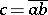, closed in the spherical metric, with endsand(including the cases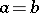and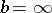), such thatbelong to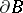, and such that the arcsubdividesinto two subdomains such that the boundary of each of them contains a point belonging toand different fromand. A sequenceof sectionsof a domainis called a chain if: 1) the diameter of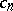tends to zero as; 2) for eachthe intersection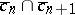is empty; and 3) any path connecting a fixed pointinwith the section,, intersects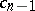. Two chainsandinare equivalent if any sectionseparates inthe pointfrom all sections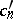, except for a finite number of them. An equivalence class of chains inis called a limit element, or prime end, of.
Letbe a prime end ofdefined by a chain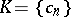, and let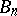be that one of the two subdomains into whichis subdivided bywhich does not contain. The set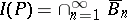is called the impression or the support of the prime end. The impression of a prime end consists of boundary points and does not depend on the selection of the chainin the equivalence class. A principal point of a prime end is a point of it to which sections of at least one of the chains defining the prime end converge. A neighbouring, or subsidiary, point of a prime end is any point of it which is not a principal point of it. Any prime end contains at least one principal point. The principal points of a prime end form a closed set. The following is the Carathéodory classification  of prime ends: Elements of the first kind contain a single principal point and no subsidiary points; elements of the second kind contain one principal point and infinitely many subsidiary points; elements of the third kind contain a continuum of principal points and no subsidiary points; elements of the fourth kind contain a continuum of principal points and infinitely many subsidiary points.
Another, equivalent, definition was given by P. Koebe . It is based on equivalence classes of paths. The principal theorem in the theory of prime ends is the theorem of Carathéodory: Under a univalent conformal mapping of a domainonto the unit disc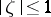there is a one-to-one correspondence between the points of the circle and the prime ends of, and each sequence of points ofwhich converges to a prime endbecomes a sequence of points in the unit disc which converge to a point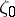,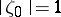, this point being the image of.